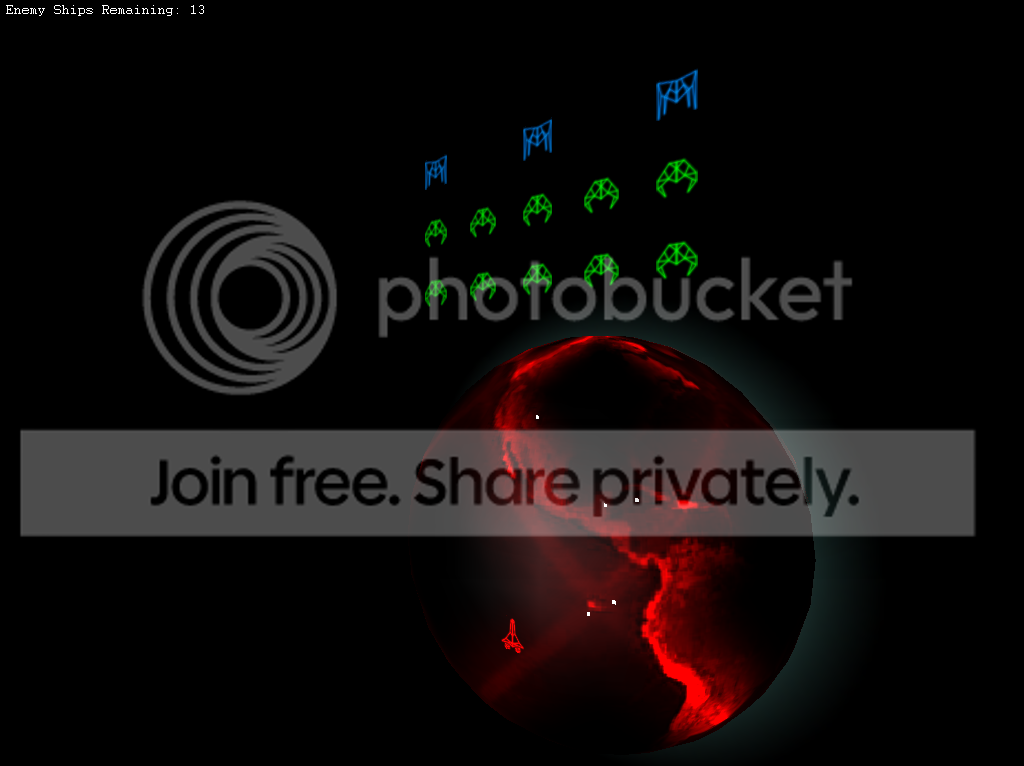-=+=- -=+=- -=+=- -=+=- -=+=- -=+=- -=+=- -=+=- -=+=- -=+=- -=+=- -=+=- -=+=- -=+=- -=+=- -=+=- -=+=- -=+=- -=+=- -=+=- -=+=- -=+=- -=+=- -=+=- -=+=- -=+=- -=+=- -=+=- -=+=- -=+=- (c) WidthPadding Industries 1987 0|648|0 -=+=- -=+=- -=+=- -=+=- -=+=- -=+=- -=+=- -=+=- -=+=- -=+=- -=+=- -=+=- -=+=- -=+=- -=+=- -=+=- -=+=- -=+=- -=+=- -=+=- -=+=- -=+=- -=+=- -=+=- -=+=- -=+=- -=+=- -=+=- -=+=- -=+=-### Scherererer

View Profile, Send Private Message
Recent Posts, Showcases, Articles, Code Snippets, Links, Wed.Workshop, Blog

### Latest Blog Entries

Scherererer# Robotics Club Competition

18th November 2009

Less than a week left!

Scherererer# Programming Team

6th September 2009

My university (the University of Central Florida) has a Programming Team. They participate nationally and internationally in competitions, every year since we started going to the South East regional we've gotten top 3. We've gotten so good that an alum (w*snip*

Scherererer# Another Brawl

3rd June 2009

I decided to start working on a super-smash brothers type game recently, just for fun. I realized that I hadn't really done any game programming in a while, but every time i learn a new coding concept, or graphical/colorspace/visual concept, I think of how*snip*

Scherererer# College Life

10th September 2008

It's Crrraaaazzzyyyyy

Scherererer# College!

21st June 2008

finally!

Scherererer25th April 2008

Now I feel soooo special...

### Latest Showcases

Scherererer23rd May 2008Another Invaders Game

Scherererer# Snooping Cat

11th March 2008I'm in ur computer, snooping ur infoz

Scherererer# NeSt Manager

16th November 2006

Network Statistic Manager